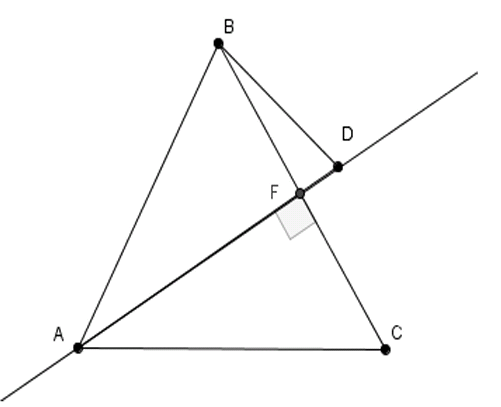# Original problem

Geometry Level 4In the figure above, $BC=BA$, $BA=AD$, and $AD\perp BC$. In degrees, find $\angle ADB +\angle BAC$.

×